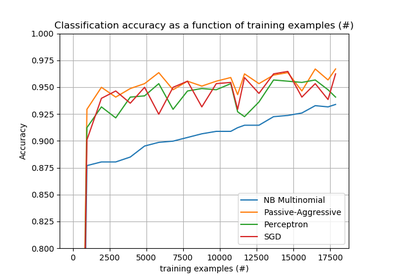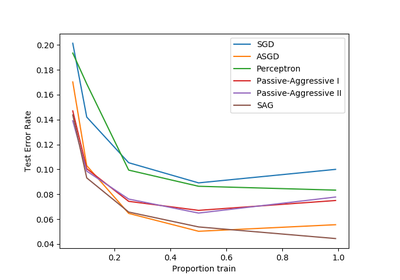# `sklearn.linear_model`.PassiveAggressiveClassifier¶

class `sklearn.linear_model.``PassiveAggressiveClassifier`(C=1.0, fit_intercept=True, max_iter=None, tol=None, shuffle=True, verbose=0, loss=’hinge’, n_jobs=1, random_state=None, warm_start=False, class_weight=None, average=False, n_iter=None)[source]

Passive Aggressive Classifier

Read more in the User Guide.

Parameters: C : float Maximum step size (regularization). Defaults to 1.0. fit_intercept : bool, default=False Whether the intercept should be estimated or not. If False, the data is assumed to be already centered. max_iter : int, optional The maximum number of passes over the training data (aka epochs). It only impacts the behavior in the `fit` method, and not the partial_fit. Defaults to 5. Defaults to 1000 from 0.21, or if tol is not None. New in version 0.19. tol : float or None, optional The stopping criterion. If it is not None, the iterations will stop when (loss > previous_loss - tol). Defaults to None. Defaults to 1e-3 from 0.21. New in version 0.19. shuffle : bool, default=True Whether or not the training data should be shuffled after each epoch. verbose : integer, optional The verbosity level loss : string, optional The loss function to be used: hinge: equivalent to PA-I in the reference paper. squared_hinge: equivalent to PA-II in the reference paper. n_jobs : integer, optional The number of CPUs to use to do the OVA (One Versus All, for multi-class problems) computation. -1 means ‘all CPUs’. Defaults to 1. random_state : int, RandomState instance or None, optional, default=None The seed of the pseudo random number generator to use when shuffling the data. If int, random_state is the seed used by the random number generator; If RandomState instance, random_state is the random number generator; If None, the random number generator is the RandomState instance used by np.random. warm_start : bool, optional When set to True, reuse the solution of the previous call to fit as initialization, otherwise, just erase the previous solution. class_weight : dict, {class_label: weight} or “balanced” or None, optional Preset for the class_weight fit parameter. Weights associated with classes. If not given, all classes are supposed to have weight one. The “balanced” mode uses the values of y to automatically adjust weights inversely proportional to class frequencies in the input data as `n_samples / (n_classes * np.bincount(y))` New in version 0.17: parameter class_weight to automatically weight samples. average : bool or int, optional When set to True, computes the averaged SGD weights and stores the result in the `coef_` attribute. If set to an int greater than 1, averaging will begin once the total number of samples seen reaches average. So average=10 will begin averaging after seeing 10 samples. New in version 0.19: parameter average to use weights averaging in SGD n_iter : int, optional The number of passes over the training data (aka epochs). Defaults to None. Deprecated, will be removed in 0.21. Changed in version 0.19: Deprecated coef_ : array, shape = [1, n_features] if n_classes == 2 else [n_classes, n_features] Weights assigned to the features. intercept_ : array, shape =  if n_classes == 2 else [n_classes] Constants in decision function. n_iter_ : int The actual number of iterations to reach the stopping criterion. For multiclass fits, it is the maximum over every binary fit.

References

Online Passive-Aggressive Algorithms <http://jmlr.csail.mit.edu/papers/volume7/crammer06a/crammer06a.pdf> K. Crammer, O. Dekel, J. Keshat, S. Shalev-Shwartz, Y. Singer - JMLR (2006)

Examples

```>>> from sklearn.linear_model import PassiveAggressiveClassifier
>>> from sklearn.datasets import make_classification
>>>
>>> X, y = make_classification(n_features=4, random_state=0)
>>> clf = PassiveAggressiveClassifier(random_state=0)
>>> clf.fit(X, y)
PassiveAggressiveClassifier(C=1.0, average=False, class_weight=None,
fit_intercept=True, loss='hinge', max_iter=None, n_iter=None,
n_jobs=1, random_state=0, shuffle=True, tol=None, verbose=0,
warm_start=False)
>>> print(clf.coef_)
[[ 0.49324685  1.0552176   1.49519589  1.33798314]]
>>> print(clf.intercept_)
[ 2.18438388]
>>> print(clf.predict([[0, 0, 0, 0]]))

```

Methods

 `decision_function`(X) Predict confidence scores for samples. `densify`() Convert coefficient matrix to dense array format. `fit`(X, y[, coef_init, intercept_init]) Fit linear model with Passive Aggressive algorithm. `get_params`([deep]) Get parameters for this estimator. `partial_fit`(X, y[, classes]) Fit linear model with Passive Aggressive algorithm. `predict`(X) Predict class labels for samples in X. `score`(X, y[, sample_weight]) Returns the mean accuracy on the given test data and labels. `set_params`(*args, **kwargs) `sparsify`() Convert coefficient matrix to sparse format.
`__init__`(C=1.0, fit_intercept=True, max_iter=None, tol=None, shuffle=True, verbose=0, loss=’hinge’, n_jobs=1, random_state=None, warm_start=False, class_weight=None, average=False, n_iter=None)[source]
`decision_function`(X)[source]

Predict confidence scores for samples.

The confidence score for a sample is the signed distance of that sample to the hyperplane.

Parameters: X : {array-like, sparse matrix}, shape = (n_samples, n_features) Samples. array, shape=(n_samples,) if n_classes == 2 else (n_samples, n_classes) : Confidence scores per (sample, class) combination. In the binary case, confidence score for self.classes_ where >0 means this class would be predicted.
`densify`()[source]

Convert coefficient matrix to dense array format.

Converts the `coef_` member (back) to a numpy.ndarray. This is the default format of `coef_` and is required for fitting, so calling this method is only required on models that have previously been sparsified; otherwise, it is a no-op.

Returns: self : estimator
`fit`(X, y, coef_init=None, intercept_init=None)[source]

Fit linear model with Passive Aggressive algorithm.

Parameters: X : {array-like, sparse matrix}, shape = [n_samples, n_features] Training data y : numpy array of shape [n_samples] Target values coef_init : array, shape = [n_classes,n_features] The initial coefficients to warm-start the optimization. intercept_init : array, shape = [n_classes] The initial intercept to warm-start the optimization. self : returns an instance of self.
`get_params`(deep=True)[source]

Get parameters for this estimator.

Parameters: deep : boolean, optional If True, will return the parameters for this estimator and contained subobjects that are estimators. params : mapping of string to any Parameter names mapped to their values.
`loss_function`

DEPRECATED: Attribute loss_function was deprecated in version 0.19 and will be removed in 0.21. Use `loss_function_` instead

`partial_fit`(X, y, classes=None)[source]

Fit linear model with Passive Aggressive algorithm.

Parameters: X : {array-like, sparse matrix}, shape = [n_samples, n_features] Subset of the training data y : numpy array of shape [n_samples] Subset of the target values classes : array, shape = [n_classes] Classes across all calls to partial_fit. Can be obtained by via np.unique(y_all), where y_all is the target vector of the entire dataset. This argument is required for the first call to partial_fit and can be omitted in the subsequent calls. Note that y doesn’t need to contain all labels in classes. self : returns an instance of self.
`predict`(X)[source]

Predict class labels for samples in X.

Parameters: X : {array-like, sparse matrix}, shape = [n_samples, n_features] Samples. C : array, shape = [n_samples] Predicted class label per sample.
`score`(X, y, sample_weight=None)[source]

Returns the mean accuracy on the given test data and labels.

In multi-label classification, this is the subset accuracy which is a harsh metric since you require for each sample that each label set be correctly predicted.

Parameters: X : array-like, shape = (n_samples, n_features) Test samples. y : array-like, shape = (n_samples) or (n_samples, n_outputs) True labels for X. sample_weight : array-like, shape = [n_samples], optional Sample weights. score : float Mean accuracy of self.predict(X) wrt. y.
`sparsify`()[source]

Convert coefficient matrix to sparse format.

Converts the `coef_` member to a scipy.sparse matrix, which for L1-regularized models can be much more memory- and storage-efficient than the usual numpy.ndarray representation.

The `intercept_` member is not converted.

Returns: self : estimator

Notes

For non-sparse models, i.e. when there are not many zeros in `coef_`, this may actually increase memory usage, so use this method with care. A rule of thumb is that the number of zero elements, which can be computed with `(coef_ == 0).sum()`, must be more than 50% for this to provide significant benefits.

After calling this method, further fitting with the partial_fit method (if any) will not work until you call densify.

## Examples using `sklearn.linear_model.PassiveAggressiveClassifier`¶Out-of-core classification of text documentsComparing various online solversClassification of text documents using sparse features